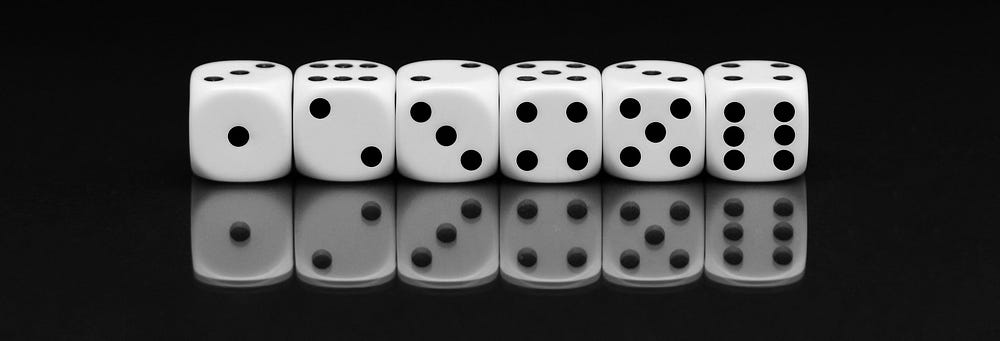# Probability Must-Haves

Original article was published on Artificial Intelligence on Medium# Understanding Random Events

Let’s say that you have a random likelihood that a user will click on a call-to-action(I’ll call it a CTA from here on out, but this is anytime you invite the reader to buy, shop, give an email, etc.) within your application. Once they have clicked the call-to-action there is a subsequent likelihood that they will press the submit button to send along their email information (in this case).

Now let’s assign some probabilities… To click the CTA: 50% likelihood and the submit button: 10% likelihood.

Now the question we have is that before either of these events takes place, what is the likelihood that BOTH will occur?

# Revisiting the Classic Probability Example: Dice

Now you may have seen this before, so at the very least this serves as an excellent refresher.

To start out, let’s answer the following question: what is the probability of rolling two subsequent 6’s.

For your first roll, you can roll the die each of the following ways: 1–2–3–4–5–6. In total, you have six options.

Obvious? yes yes, but now here’s where we think a bit more critically about this. If you roll a 1, what is your next option? Well, it’s any of the following numbers: 1–2–3–4–5–6. If you had rolled a 2, you’d have the same 6 total options for the second roll. In this scenario, you would have 6 options for your second throw. In total, this gives us 36 (6*6) different outcomes.

So back to the question… what is the likelihood that you might roll two 6’s in a row?

Well if we roll a die, the likelihood of the first landing on 6 is 1/6. Once that has happened, we have a likelihood of 1/6 again. In all of the 36 different combinations of two rolls, there is only one in which a 6 is rolled twice in a row. The math for this is to simply multiply the two probabilities together giving you a 1/36 likelihood that you would roll two 6s in a row.

# Back to the App Example

Let’s apply what we just learned to our original example. If there is a 1/2 chance of a user hitting the CTA and a 1/10 chance of them hitting the submit button; then we can multiply our probabilities together to say that there is a 1/20 or 5% chance that they will hit both buttons.

# Theory Aside, Let’s Write Some Code

To preface here; if you haven’t seen my medium post on statistical inference I detail the `rbinom` function: https://towardsdatascience.com/an-introduction-to-binomials-inference-56394956e1a4. To give you the quick version of the significance of this, you use this function to simulate randomly occurring binomial events.

# Let’s Require Both Using…. And

We have 5000 draws of the event, with their corresponding probabilities. We will see 5000 draws of either 1 or 0. When we specify the `&`, we are effectively saying that both must be true. When we take an average of the occurrences of both being true gives us the probability that both would occur.

`CTA <- rbinom(5000, 1, .5) SUBMIT <- rbinom(5000, 1, .1) mean(CTA & SUBMIT)`